## Quiz 6 : Annual Equivalent-Worth AnalysisLooking for Economics Homework Help?# Quiz 6 : Annual Equivalent-Worth Analysis

The annual equivalent worth is a criterion used to determine the value of an investment by determining equal amount of payments on yearly basis. The net present worth of cash flow is the value of future cash flows at present. In order to determine equivalent annual worth, first net present worth is to determine. The Net present worth can be calculated using the following formula: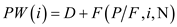Where, The present worth is PW (i). The present worth of the lump sum of money be P. The future value of the sum of money be F. The initial cash flow in the series is D. The annual amount of a cash flow series be A. The interest rate be i. The number of terms that the money be N. The formula to calculate equivalent annual worth is given below: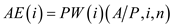Where, The present worth is PW (i). The present worth of the lump sum of money be P. The annual amount of a cash flow series be A. The interest rate be i. The number of terms that the money be N. The net present worth of the cash flow at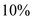is calculated as follows: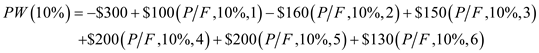Use the table in Appendix B from the text book, to get the factor values. Substitute the values in the above expression and solve as follows:Thus, the present worth is \$105.54. Now the equivalent present worth is to determine. Substitute the known value in the formula as follows: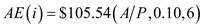Next use Appendix B in the textbook to get the factor value. The value applicable here is 0.2296. Substitute the value and solve as follows: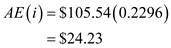Hence, the annual equivalent worth for the cash flow is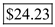per year.

The sixth chapter of the textbook focuses on various ways that a person can determine the equivalent annual worth for a given project as well as other things like determining the unit cost or unit profit as well as a life-cycle cost analysis amongst other things. For the case study in question, we have absorption chillers as the item of focus. These units provide cooling to buildings by using heat. It is one of the most cost effective ways to provide air conditioning to larger buildings that are also environmentally friendly. Additional information provided in the text shows that the annual savings of using one of these units is approximately \$52,740.00 for a facility that is of normal size (usually more than 50,000 square feet and more than likely multiple floors). Regarding the problem at hand, suppose one plan to install a chiller unit and have an assumption that it would run continuously for 10 years (as it would be its useful life). What would be the maximum amount that should be invested in a situation like this with the capacity described above Assume an interest rate of 10.00% for the situation at hand and the energy costs are projected to increase by 10.00% annually as well. In the situation that we have here, a lot of the leg work is already done for us which are a great benefit in our favor. The situation that we have here needs to be done in two (2) steps. The first step is to find the present worth of the annual savings. When this done, the following formula and calculations below are needed to get this first part out of the way: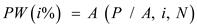….. (1) Where… - PW(i) = the present worth cost for a cash flow series, - P = present value of the sum of money, - A = the annualized costs for the equipment/cash flow in question, - i = interest rate (which is known in this case), and - n = number of terms that the money is for. We begin the calculations are shown below to get us going: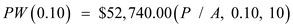The next thing that should be done here is to mosey on over to the back of the textbook to get the values for the factor that is shown above. Note that the factors that are applicable to the problem are found in the fifth column for the factor needed here. The values are shown below and the remainder of the calculations is presented: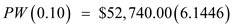PW (0.10) = \$324,066.20 Thus, the present worth for the cash flow series assuming the interest rate of 10.00% and a 10 year period is approximately \$324,066.20. The second thing that one would need to do in order to get the other present worth factor done properly here is to find out what it would be with the 10.00% gradient applied for the increased energy costs. This is shown below for one to see and solve: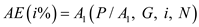…… (2) Where… - P = the present worth of the sum of money in this situation, - A = annual amount placed in the account, - G = the gradient that the cash flows are changing over time, - i = interest rate (which is known in this case), and - N = number of terms that the money is for. Given the situation above, we now need to apply the values that were mentioned at the top of the problem. When we do this, notice that this isn't your ordinary problem that one would have to solve here but it is one that is doable if done properly: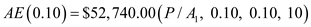The best way that one could tackle the problem is to apply a formula in Microsoft Excel which can help with calculating the equivalence between the two (2) options. It is important to note that that when the interest rate and gradient are equal, we would need to apply the following formula below. This is much easier than the pair being different values: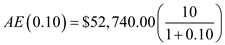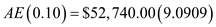AE (0.10) = \$479,454.55 Thus, the annual equivalent costs for the gradient that is applied to the situation is approximately \$479,454.55.

The Annual Equivalent Cost method is used when the costs are only involved in the particular project. Under this method, revenues earned must recover two types of cost that is capital cost and operating cost. Moreover, the annual cost is regarded as the cost with respect to ownership, operating as well as maintaining of assets. As per the problem, a cash flow diagram is given in which the revenue at present is \$20,000 and the cost is \$5,000 during first 2 periods then it increases by \$3,000 every year till sixth year. The rate of return is 13%. Write the formula to calculate the equivalent annual worth for the cash flow series over 6-year period at interest rate 12% as follows: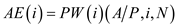Write the formula to calculate the present worth as follows: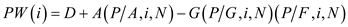Here, The present worth is PW (i). The present worth of the lump sum of money be P. The future value of the sum of money be F. The gradient that is present in the cash flow is G. The initial cash flow in the series is D. The annual amount of a cash flow series be A. The interest rate be i. The number of terms that the money be N. Calculate the annual equivalent cost as follows: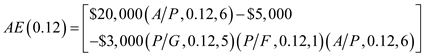The values for the factor can be taken from the appendix. Substitute the factor values and solve as follows:Thus, the annual equivalent cost for the cash flow is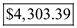.

There is no answer for this question